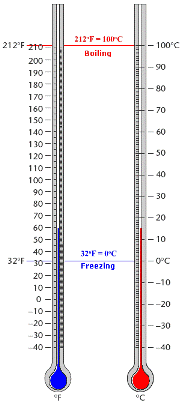# Temperature

Temperature is the measure of hotness or coldness of an object. Temperature can be measured in metric units or in U.S. customary units. We can use a thermometer that has both a Celsius ( $°C$ ) and a Fahrenheit ( $°F$ ) scale to measure the temperature.

The following table shows the different temperatures on Celsius ( $°C$ ) and a Fahrenheit ( $°F$ ) .

 Temperature Fahrenheit ( $°F$ ) Celsius ( $°C$ ) Normal Human Body Temperature $98°F$ $37°C$ Room Temperature $68°F$ $20°C$ Water Boils $212°F$ $100°C$ Water Freezes $32°F$ $0°C$

Metric Units

In metric unit, temperature is measured in degrees Celsius ( $°C$ ). The Celsius scale is also called the centigrade scale since it's divided into $100$ degrees. The Swedish astronomer Anders Celsius who developed Celsius temperature scale.

U.S. Customary Units

In the customary unit, temperature is measured in degrees Fahrenheit ( $°F$ ). The physicist Gabriel Daniel Fahrenheit who named Fahrenheit ( $°F$ ) in $1724$ .The thermometers on the above figure show the water freezes at $0°C$ or $32°F$ and boils at $100°C$ or $212°F$ .

To convert temperatures from Fahrenheit to Celsius, use the formula $C=\frac{5}{9}\left(F-32\right)$ and for Celsius to Fahrenheit is $F=\frac{9}{5}C+32$ .

For Example, the lowest recorded temperature in Oklahoma is $-31°F$ on February $9$ , $2011$ . Find the temperature in degree Celsius.

The expression to convert a temperature in Fahrenheit to degree Celsius is $C=\frac{5}{9}\left(F-32\right)$ .

To convert $-31°F$ to degree Celsius, first replace $F$ with $-31$ .

$\begin{array}{l}\frac{5}{9}\left(F-32\right)=\frac{5}{9}\left(-31-32\right)\\ =\frac{5}{9}\left(-63\right)\end{array}$

Next, multiply.

$\frac{5\left(-63\right)}{9}=\frac{-315}{9}$

Now, divide.

$=-35$

Therefore, the temperature in degrees Celsius is $-35°C$ .

Note : The Kelvin( $K$ ), a unit of thermodynamic temperature, is the $\frac{1}{273.16}$ of the absolute temperature of the triple point of water.